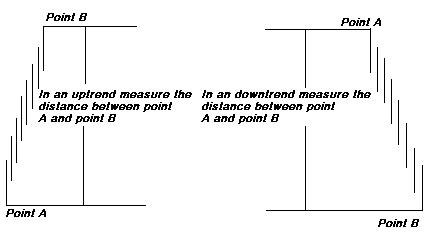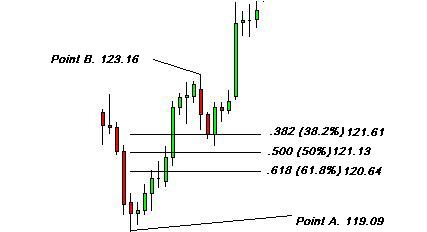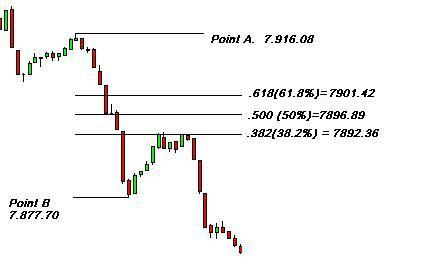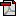Home | Previous | Next Lesson

This Lesson Can Be Printed - See Instructions Below.

# Fibonacci - The Most Powerful Indictor

Of all the trading tools I have ever used by far my favorite tool for analysis is Fibonacci studies. This is such a large and complex study that I shall not even pretend to be an expert in this area. Instead I would like to demonstrate how I apply a limited knowledge of the subject to my trading.

We shall only be discussing three primary Fibonacci ratios and not minor ratios, ovals, arcs, bands or the time axis.

 First Some History Leonardo Fibonacci da Pisa was born around 1170 the son of a city official and merchant. He became a prominent mathematician and is credited with the discovery of what we now call the Fibonacci series. After a trip to Egypt he published his now famous Liber Abacci (Book of Calculation) in which amongst other things he comes up with the sequence of numbers. 1,1,2,3,5,8,13,21,34,55,89>>On to infinity If you add one of the numbers in the sequence to the number before it you get the next number in the sequence e.g. 3+5=8 and so on.

After the first few numbers in the sequence if you measure the ratio of any number to that of the next higher number you get .618 to 1 e.g. 34 divided by 55 equals 0.618. The further along the sequence you go the closer to phi you will get.

If you measure the ratio between alternative number you get .382 e.g. 34 divided by 89 = 0.382 and that's about as far into the explanation as I care to go. As a trader you don't need to know any of this. All you need to know is if your charting software has Fibonacci capabilities. If it does then that will work everything out for you.

The three Fibonacci ratios we shall use are .382, .500 and .618 and how we can use them in our day to day trading.

In an uptrend measure the distance between point A and point B and in a downtrend measure the distance between point A and point B where point A will always be the lowest recent point in an uptrend and the highest recent point in a downtrend.In the example below you can see a chart of the daily JPY/USD. Point A is 119.09 and Point B is 123.16. If you calculate the 38.2% retracement you get 121.61, the 50% retracement is 121.13 and the 61.8% retracement is 120.64. For example. The difference between 119.06 and 123.16 is 4.07. If you calculate 38.2% of 4.07 you get 1.55. If you then take 1.55 from 123.16 (Point B) you get the 38.2% retracement of 121.61. You can use the same principal for the other retracement levels.In our next example of the 1-minute Dow Jones Point A is 7.916.08 and point B is 7.877.70. If you calculate the 38.2% retracement you get 7892.36, the 50% retracement is 7896.89 and the 61.8% retracement is 7901.42. For example. The difference between 7.916.08 and 7877.70 is 38.38, if you calculate 61.8% of that you get 23.72. If you then take 23.72 and add it to Point B of 7.877.70 you get 7901.42 the 61.8% retracement. The only difference between the downtrend and the uptrend is that you add your calculations to Point B and in the uptrend you subtract from Point B.So how can we use all this information? Well, you would be amazed just how many times a security will find support or resistance at Fibonacci levels. I think a large part of this may be that so many traders use this technique in their analysis that it is a self-fulfilling prophesy and part because it falls into the natural order of the market.

I apply this technique by first identifying a trend in the market I am following. As soon as I can see that there is going to be a retracement I them calculate my retracement levels. I then enter at the 38.2% retracement level and place my stop loss behind the 61.8% retracement level.

If I feel the difference between the 38.2% and 61.8% level is to great a risk I drop down a time frame and use the same technique but get a much tighter stop.

In our next lesson I shall show you how to work out targets with Fibonacci, you will then have a logical place to enter the market, a logical place to put your stop and a logical target.

Good Trading

Mark McRae

PS. Don't for get to check out our bookstore at http://www.surefire-trading.com/

Information, charts or examples contained in this lesson are for illustration and educational purposes only. It should not be considered as advice or a recommendation to buy or sell any security or financial instrument. We do not and cannot offer investment advice. For further information please read our disclaimer.To PRINT or save a copy of this lesson in PDF format simply click the PRINT link. This will open the lesson in a PDF format which, you can then PRINT. If you are unfamiliar with PDF or don't have a FREE copy of Arobat Reader see instructions.